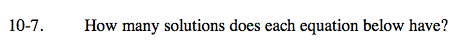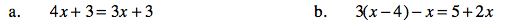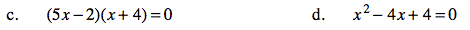### Home > AC > Chapter 10 > Lesson 10.1.1 > Problem10-7

10-7.Solve the equation for x.

x = 0

1 solution

Follow the steps in part (a).

Are there any solutions to this equation?One of the factors must be equal to zero.

2 solutions

Factor the equation.

Follow the steps in part (c).